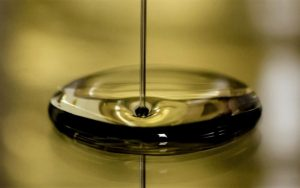Chemistry

# Newtonian fluid

A fluid is said to be Newtonian if its viscosity, which is the measure or ability of a fluid to resist flow, only varies as a response to changes in temperature or pressure. A Newtonian fluid will take the shape of its container. Under conditions of constant temperature and pressure, the viscosity of a Newtonian fluid is the proportionality constant, or the relationship between the shear force formed in the fluid to resist the flow and the shear velocity applied to the fluid to induce the flow; the viscosity is the same for all shear velocities applied to the fluid. Water, sugar solutions, glycerin, silicone oils, light hydrocarbons, air and other gases are all examples of Newtonian fluids. Most drilling fluids are non-Newtonian fluids.Related Topics

Non-Newtonian fluid

## What is Newtonian fluid?

The Newtonian fluid is a fluid that has a viscosity that can be considered as constant over time with a curve that shows the relationship between the stress against the rate of deformation is linear.

## Characteristics of Newtonian fluid

The main characteristics of the Newtonian fluid are as follows:

• Newtonian fluids have no elastic properties.
• They are incomprehensible, isotropic and unreal.
• Viscosity depends on temperature.
• Viscosity also depends on the different pressures at which it is found.
• When they are at a fixed temperature, their viscosity does not change and remains constant.
• It is said that these fluids have a normal behavior, in which there is very little viscosity, and this does not vary with forces that are applied on it.
• As the temperature increases in a fluid, its viscosity decreases.
• The viscosity of the liquid is inversely proportional to the increase in its temperature.
• The Newtonian fluid was named by Isaac Newton, who described it as a viscous flow.
• They comply with Newton’s law of viscosity.

## Classification of Newtonian fluids

Newtonian fluids can be classified depending on the relationship between the shear stress applied to the flow and the speed of deformation resulting from this stress. Fluids in which the shear stress is directly proportional to the deformation rate are called Newtonian fluids.

## Viscosity of Newtonian fluid

The study carried out with respect to the different types of viscosity of liquids is based on studying the different speed profiles that are generated under certain types of conditions, always first considering the Newtonian idea, in which viscosity is a constant that will depend mainly on temperature and to a lesser extent will depend on pressure.

## Temperature

In a fluid that has already been considered a Newtonian fluid, the viscosity will depend solely on the temperature and the differences that are present. As the temperature increases, the viscosity of the fluid will decrease. In other words, the viscosity of the fluids is inversely proportional to the increase in temperature.

## Examples of Newtonian fluid

There are a large number of fluids that have the necessary characteristics to be considered as a Newtonian fluid, in addition to behaving like a Newtonian fluid under normal conditions of pressure and temperature. Among them we can mention the following:

SAE 30 oil, which is a motor and transition oil generally used in automobiles.

All those foods that show ideal flow characteristics, some of them of great physiological importance, such is the case of water, milk of any type of animal that can produce it, and drinks that are taken as refreshments.

All those solutions that are made with sugars, therefore, all types of carbonated beverages, alcoholic beverages that do not contain long chain molecules, corn syrups and some types of honey fall into this classification.

• Hydrogen
• Gasoline
• Air
• The different types of oils that exist.
• Ethyl alcohol.
• Mercury.
• Glycerin
• Benzene
• All those liquid compounds based on oils.
Written by Gabriela Briceño V.